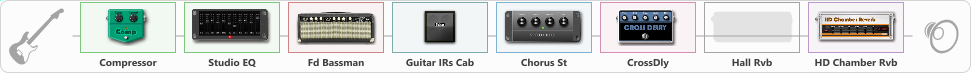# Killer Of Giants

Discussion in 'ToneLib-GFX presets' started by davidtaylors, Nov 12, 2021.

1. ### davidtaylorsWell-Known Member

Killer Of Giants

Preset name: Killer Of Giants

Bridge second position on humbucker

Effects chain:Effect: "Compressor" (Dynamics / Filter), active - "yes"
{
"Sense" = 23
"Level" = 63
}

Effect: "Studio EQ" (Dynamics / Filter), active - "yes"
{
"31 Hz" = 6
"62 Hz" = 8
"125 Hz" = 5
"250 Hz" = 3
"500 Hz" = 8
"1 kHz" = -15
"2 kHz" = 7
"4 kHz" = 9
"8 kHz" = 4
"16 kHz" = 0
"Level (dB)" = 0
}

Effect: "Fd Bassman" (Amp simulators), active - "yes"
{
"Gain" = 31
"Bass" = 74
"Middle" = 61
"Treble" = 80
"Presence" = 61
"Master" = 54
"Level (dB)" = 0
}

Effect: "Guitar IRs Cab" (Cabinets), active - "yes"
{
"Model" = Fender Twin Rev (2x12")
"Mic Position" = Center
"Mic Distance" = Far
"Low Cut (Hz)" = 60
"Hi Cut (kHz)" = 20.0
"Mix" = 100
"Level (dB)" = 0
}

Effect: "Chorus St" (Modulation / Sfx), active - "yes"
{
"Speed" = 3.1
"Depth" = 41
"Center" = 2.6
"Mix" = 100
}

Effect: "CrossDly" (Delay), active - "yes"
{
"Time" = 740
"Feedback" = 75
"Tone" = 48
"Sens" = 3
"Mix" = 70
}

Effect: "Hall Rvb" (Reverberation), active - "no"
{
"Time" = 9.4
"PreDelay" = 61
"LoDamp" = 49
"HiDamp" = 17
"Mix" = 44
}

Effect: "HD Chamber Rvb" (Reverberation), active - "yes"
{
"Time" = 8.2
"PreLPF" = 79
"PreDelay" = 921
"HiDamp" = 21
"LoGain" = -2.1
"Mix" = 37
}

Note: You will need to download and install the ToneLib-GFX software to use the preset.

#### Attached Files:

• ###### Killer_Of_Giants.tlgfx
File size:
866 bytes
Views:
2,100
Regina, ppv, Alexey Borovkov and 2 others like this.